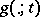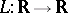# Edge detection

An early processing stage in image processing and computer vision, aimed at detecting and characterizing discontinuities in the image domain.

The importance of edge detection for early machine vision is usually motivated from the observation that under rather general assumptions about the image formation process, a discontinuity in image brightness can be assumed to correspond to a discontinuity in either depth, surface orientation, reflectance, or illumination. In this respect, edges in the image domain constitute a strong link to physical properties of the world. A representation of image information in terms of edges is also compact in the sense that the two-dimensional image pattern is represented by a set of one-dimensional curves. For these reasons, edges have been used as main features in a large number of computer vision algorithms.

A non-trivial aspect of edge-based analysis of image data, however, concerns what should be meant by a discontinuity in image brightness. Real-world image data are inherently discrete, and for a function defined on a discrete domain, there is no natural notion of "discontinuity" , and there is no inherent way to judge what are the edges in a given discrete image.

An early approach to edge detection involved the convolution of the imageby a Gaussian kernel, followed by the detection of zero-crossings in the Laplacian response [a1] (cf. also Scale-space theory). However, such edge curves satisfyinggive rise to false edges and have poor localization at curved edges.

A more refined approach is the notion of non-maximum suppression [a2], [a3], [a4], where edges are defined as points at which the gradient magnitude assumes a local maximum in the gradient direction. In differential-geometric terms, such edge points can be characterized as points at which [a5]:

i) the second-order directional derivative in the gradient direction is zero; and

ii) the third-order directional derivative in the gradient direction is negative.

In terms of partial derivatives, for a two-dimensional imagethis edge definition can be written asAgain, the computation of discrete derivative approximations is preceded by smoothing the imagewith a Gaussian kernel, and the choice of different standard deviations of the Gaussian kernel gives rise to edges at different scales (see Scale-space theory or [a5]). While other choices of linear smoothing kernels have also been advocated, their shapes can often be well approximated by Gaussians [a3], [a6], [a7].

Other approaches to edge detection involve the thresholding of edge strength measures, the computation of intensity derivatives from local least squares fitting, and functional minimization (see also [a8]).

A subject which has been given large attention during the 1990s is the replacement of the linear smoothing operation by a non-linear smoothing step, with the goal of avoiding smoothing across object boundaries [a9], [a10].